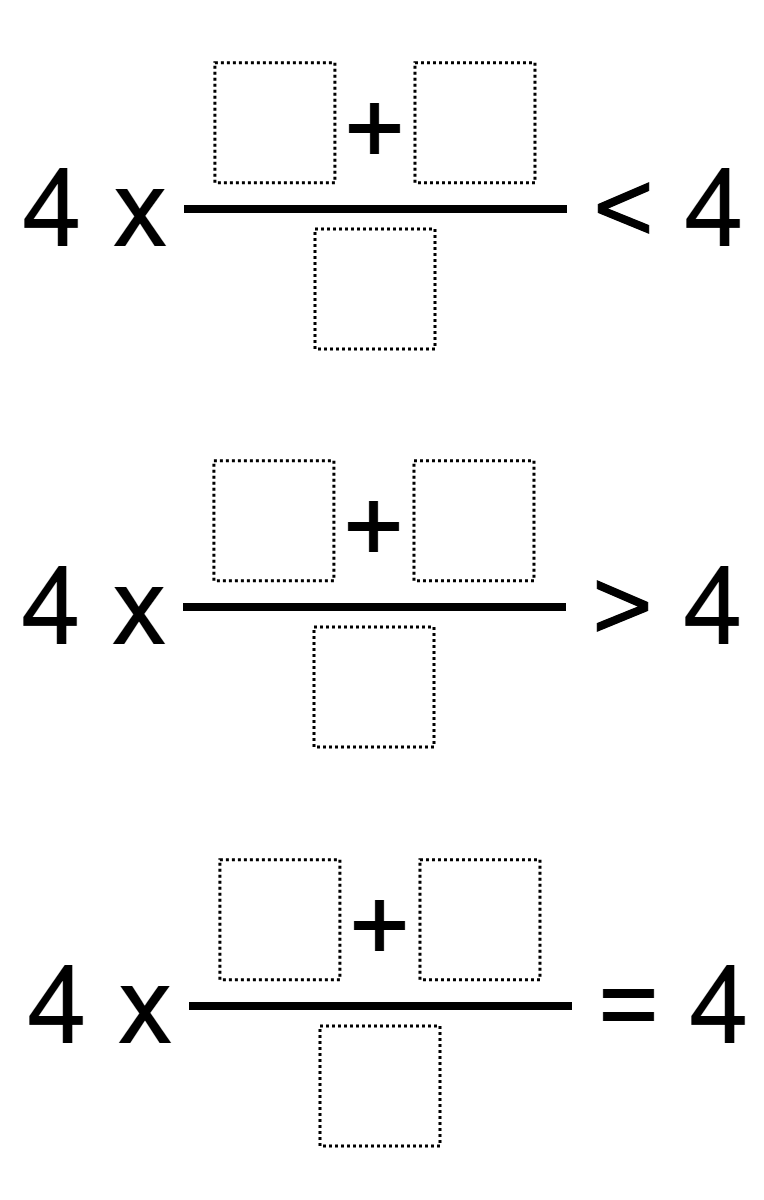Home > Grade 5 > Fraction Equivalence

# Fraction Equivalence

Directions: Using the digits 1 to 9 at most one time each, fill in the boxes to create a fraction that correctly completes each statement.### Hint

What is the result of multiplying a whole number by a proper fraction?
What is the result of multiplying a whole number by an improper fraction?

### Answer

Multiple answers:

ex. (1+3)/9 < 4, (6+2)/8 = 4, (5+7)/4 > 4

Source: Ian Kerr

## Multiplying Decimals to Make a Whole Number Product

Directions: Using the digits 1 to 9 at most one time each, fill in the …